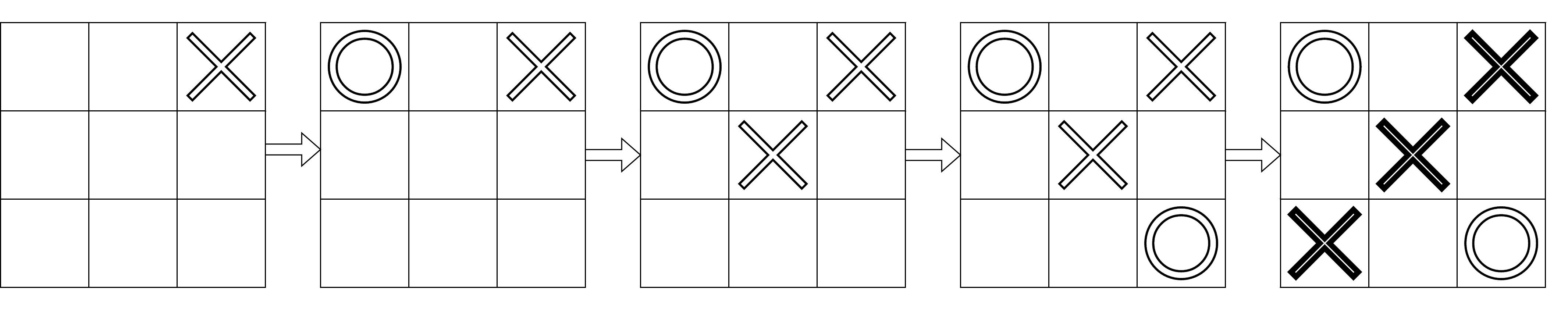# Tic-Tac-Toe Winner

Posted: 26 Feb, 2021
Difficulty: Easy

## PROBLEM STATEMENT

#### Example :

``````n = 5, moves = {{0,2}, {0,0}, {1,1}, {2,2}, {2,0}}
``````#### Note :

``````1. The array ‘moves’ doesn’t contain any repeating positions, and all positions are valid.
2. The array ‘moves’ follows all the rules of tic-tac-toe.
3. You do not need to print anything; it has already been taken care of. Just implement the function
``````

#### Input format :

``````The first line of input contains an integer ‘T’ denoting the number of test cases.

The first line of each test case contains an integer ‘N’ denoting the size of the array ‘moves’.

The next ‘N’ lines of each test case contain two space-separated integers denoting a tuple of the array ‘moves’.
``````

#### Output format :

``````For each test case, if there is a winner, print their name, i.e., ‘player1’ or ‘player2’. Otherwise, depending on the grid’s final state, print ‘uncertain’ or ‘draw’ (without quotes).
``````

#### Constraints :

``````1 <= T <= 1000
1 <= N <= 9
| moves[i] | = 2
0 <= moves[i][j] <= 2

Where ‘moves[i][j]’ represents elements in the array ‘moves’.

Time limit: 1 second
``````Approach 1

Create a 2-D array ‘grid’ of size ‘3 x 3’ with each element set to 0. Use this array ‘grid’ to store the state of the actual grid. We know that  ‘player1’ makes all the even moves (0-based indexing), and ‘player2’ makes all the odd moves because ‘player1’ always plays first, and the players take alternate turns. For a move by ‘player1’, mark the ‘grid’ position as 1. For a move by ‘player2’, mark the ‘grid’ position as 4. Check if any row, column, or diagonal has three same characters and return the appropriate answer.

• Create a 2-D array ‘grid’. Set all elements of ‘grid’ to 0.
• Run a loop where ‘i’ ranges from 0 to ‘n-1’ and ‘i’ increments by 2 after each iteration. Set ‘grid[ moves[i] ][ moves[i] ] = 1’.
• Run a loop where ‘i’ ranges from 1 to ‘n-1’ and ‘i’ increments by 2 after each iteration. Set ‘grid[ moves[i]][ moves[i]] = 4’.
• Initialize ‘diagOneSum’ and ‘diagTwoSum’ with the value 0. Use these two variables for the sum of the first and second diagonal, respectively.
• Run a loop where ‘i’ ranges from 0 to 2.
• Initialize ‘rowSum’ and ‘colSum’ with the value 0. Use these two variables for the sum of the ‘i-th’ row and column, respectively.
• Run a loop where ‘j’ ranges from 0 to 2.
• Compute ‘rowSum += grid[i][j]’.
• Compute ‘colSum += grid[j][i]’.
• If (‘rowSum’ equals 3 or ‘colSum’ equals 3) return ‘player1’ .
• If (‘rowSum’ equals 12 or ‘colSum’ equals 12) return ‘player2’.
• Compute ‘diagOneSum += grid[i][i]’ and ‘diagTwoSum += grid[i][2-i]’.
• If (‘diagOneSum’ equals 3 or ‘diagTwoSum’ equals 3) return ‘player1’.
• If (‘diagOneSum’ equals 12 or ‘diagTwoSum’ equals 12) return ‘player2’.
• At this step, we know that there is no winner. So, if n equals 9, return ‘draw’ else return ‘uncertain’.